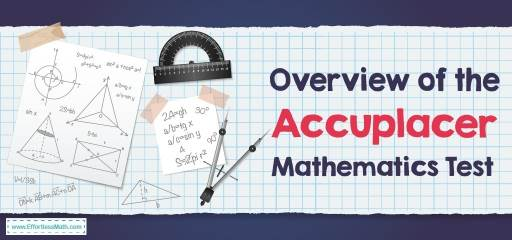# Overview of the ACCUPLACER Math TestThe ACCUPLACER is the name of a set of subject tests created by the College Board to assess a college student’s readiness for the knowledge and skills needed to succeed in introductory-level College courses. Colleges and universities offer various ACCUPLACER tests for eligible students to determine what courses students should take when starting university.

The ACCUPLACER test is an untimed, multi-choice computer-compatible test that allows you to work at a speed and level that is comfortable for both you and your abilities. The ACCUPLACER is divided into the following subtests:

• Arithmetic
• Quantitative Reasoning, Algebra, and Statistics
• Writing

The math-related subsections of the ACCUPLACER include:

• Arithmetic
• Quantitative Reasoning, Algebra, and Statistics (QAS)
• Advanced Algebra and Functions (AAF)

## How is the structure of the ACCUPLACER Arithmetic Subtest?

In the ACCUPLACER Arithmetic subtest, you will be presented with 20 questions that aim to assess your understanding of fundamental arithmetic through the following categories of knowledge and skills:

• Decimal Operations (3-5 questions)
• Fraction Operations (3-5 questions)
• Number Comparisons and Equivalents (3-5 questions)
• Whole Number Operations (3-5 questions)
• Percent (3-5 questions)

The Arithmetic subtests include:

computation (subtraction, addition, multiplication, division), comparing and ordering values in different formats, estimating and rounding, ordering operations, and recognizing equivalent values in forms (fractions, decimals, mixed numbers, percentages).

## How is the structure of the ACCUPLACER Quantitative Reasoning, Algebra, and Statistics (QAS) Subtest?

The ACCUPLACER QAS test questions focus on a wide range of mathematical topics, including computing with rational numbers, applying ratios and proportional reasoning, and linear equations. It tests math skills more than arithmetic. This test asks you to answer 20 multiple choice questions that deal with different content areas, including:

• Algebraic Expressions (2-3 questions)
• Descriptive Statistics (1-3 questions)
• Exponents (2-3 questions)
• Geometry Concepts for Algebra I (1-2 questions)
• Geometry Concepts for Pre-Algebra (1-2 questions)
• Linear Applications and Graphs (2-4 questions)
• Linear Equations (2-4 questions)
• Probability and Sets (1-3 questions)
• Ratio and Proportional Relationships (3-4 questions)
• Rational numbers (1-3 questions)

## How is the structure of the ACCUPLACER Advanced Algebra, and Functions (AAF) Subtest?

The ACCUPLACER Advanced Algebra and Functions (AAF) test assesses students’ ability to deal with a variety of equations and mathematical functions, as well as basic geometry and trigonometry.

Like other math tests, this test consists of a total of 20 multiple-choice questions. The specific content areas in the AAF test, the skills and knowledge assessed in those content areas, and the number of possible test questions related to each content area are as follows:

• Exponential and Logarithmic Equations (1-3 questions)
• Factoring (1-2 questions)
• Functions (2-4 questions)
• Geometry Concepts for Algebra I (1-2 questions)
• Geometry Concepts for Algebra II (1-2 questions)
• Linear Applications and Graphs (2-3 questions)
• Linear Equations (2-3 questions)
• Polynomial Equations (1-3 questions)
• Radical and Rational Equations (1-3 questions)
• Trigonometry (1-3 questions)

Of the three ACCUPLACER math tests, AAF is the most challenging. This is because it tests you in more advanced skills and concepts!

## Is theACCUPLACER Mathematics test hard?

The ACCUPLACER math test is quite difficult because it is a computer-compatible test. In other words, the more questions you receive correctly, the more difficult the questions for the next questions will be.

## Can you use a calculator on the ACCUPLACER test?

In the math section of the test, test takers are not allowed to use a personal calculator, but the test center provides an on-screen calculator for students for some questions.

## Do you get a formula sheet on theACCUPLACER test?

The ACCUPLACER provides math formulas for some questions. Formulas are provided for you to focus on application instead of memorizing formulas. However, this test does not provide all the math formulas you need to know for the test. It means that you should recall many mathematical formulas in ACCUPLACER.

## How is theACCUPLACER math test scored?

The ACCUPLACER score range for Quantitative Reasoning, Algebra and Statistics (QAS), Arithmetic, Advanced Algebra and Functions (AAF) is 200-300.

## The Best Books to Ace the Accuplacer Math Test

### What people say about "Overview of the ACCUPLACER Math Test - Effortless Math: We Help Students Learn to LOVE Mathematics"?

No one replied yet.

X
52% OFF

Limited time only!

Save Over 52%

SAVE \$40

It was \$76.99 now it is \$36.99